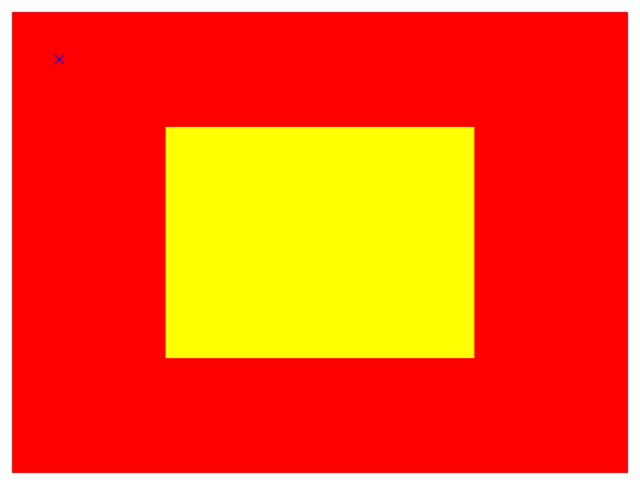Instance Method

convert(_:to:)

Converts the point from the receiver’s coordinate system to the specified layer’s coordinate system.

Parameters

p

A point specifying a location in the coordinate system of l.

l

The layer into whose coordinate system p is to be converted. The receiver and l must share a common parent layer. This parameter may be nil.

Return Value

The point converted to the coordinate system of layer.

Discussion

If you specify nil for the l parameter, this method returns the original point added to the layer's frame's origin.

Listing 1 shows code that creates two layers, redLayer and yellowLayer. yellowLayer is scaled so that it is half of its original size.

Figure 1 shows the two layers and an overlaid point (rendered as a blue cross) with a position of (50.0, 50.0) in the red layer's coordinate system.Figure 1 Layers with different coordinate systems

Listing 2 show how you can find the coordinates of that point in the yellow layer's coordinate system.

Mapping Between Coordinate and Time Spaces

func convert(CGPoint, from: CALayer?) -> CGPoint

Converts the point from the specified layer’s coordinate system to the receiver’s coordinate system.

func convert(CGRect, from: CALayer?) -> CGRect

Converts the rectangle from the specified layer’s coordinate system to the receiver’s coordinate system.

func convert(CGRect, to: CALayer?) -> CGRect

Converts the rectangle from the receiver’s coordinate system to the specified layer’s coordinate system.

func convertTime(CFTimeInterval, from: CALayer?) -> CFTimeInterval

Converts the time interval from the specified layer’s time space to the receiver’s time space.

func convertTime(CFTimeInterval, to: CALayer?) -> CFTimeInterval

Converts the time interval from the receiver’s time space to the specified layer’s time space